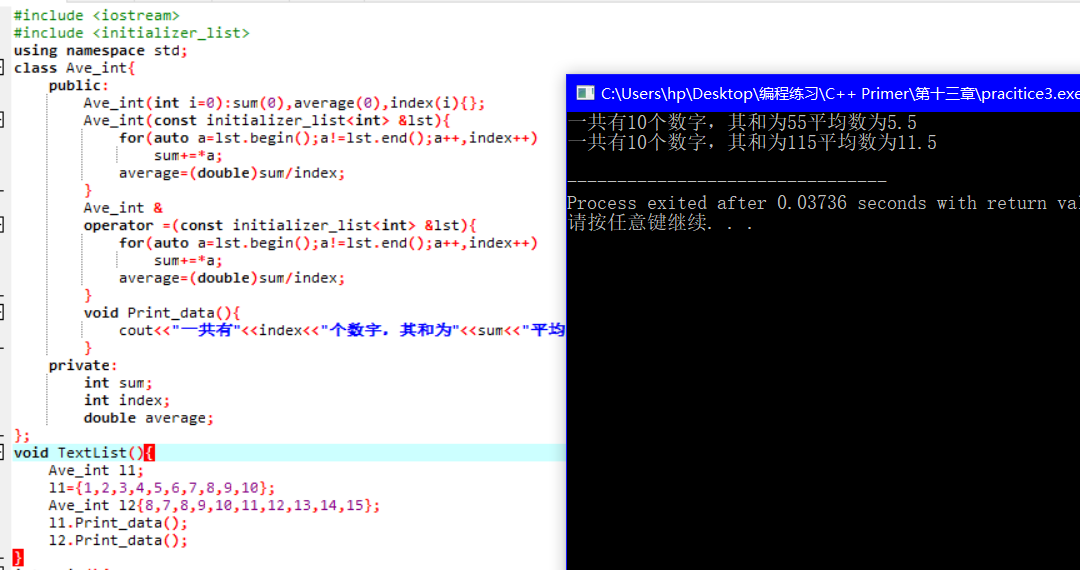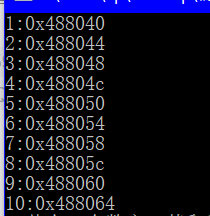简单剖析一下关于initializer_list 的实际实用

vector<string> S1{"Hello","It's","me"};

#include <iostream>
#include <initializer_list>
using namespace std;
class Ave_int{
public:
Ave_int(int i=0):sum(0),average(0),index(i){};
Ave_int(const initializer_list<int> &lst){
for(auto a=lst.begin();a!=lst.end();a++,index++)
sum+=*a;
average=(double)sum/index;
}
Ave_int &
operator =(const initializer_list<int> &lst){
for(auto a=lst.begin();a!=lst.end();a++,index++)
sum+=*a;
average=(double)sum/index;
}
void Print_data(){
cout<<"Ò»¹²ÓÐ"<<index<<"¸öÊý×Ö£¬ÆäºÍÎª"<<sum<<"Æ½¾ùÊýÎª"<<average<<endl;
}
private:
int sum;
int index;
double average;
};
void TextList(){
Ave_int l1;
l1={1,2,3,4,5,6,7,8,9,10};
Ave_int l2{8,7,8,9,10,11,12,13,14,15};
l1.Print_data();
l2.Print_data();
}

Ave_int l1;
l1={1,2,3,4,5,6,7,8,9,10};
Ave_int l2{8,7,8,9,10,11,12,13,14,15};allocator<string> alloc;
StrVec(const initializer_list<string> &lst){
auto newdata=alloc_n_copy(lst.begin(),lst.end());
elements=newdata.first;
first_free=newdata.second;
}
pair<string *, string *> StrVec::alloc_n_copy(const string *b, const string *e) {
auto data = alloc.allocate(e - b);
return { data,uninitialized_copy(b,e,data) };
}